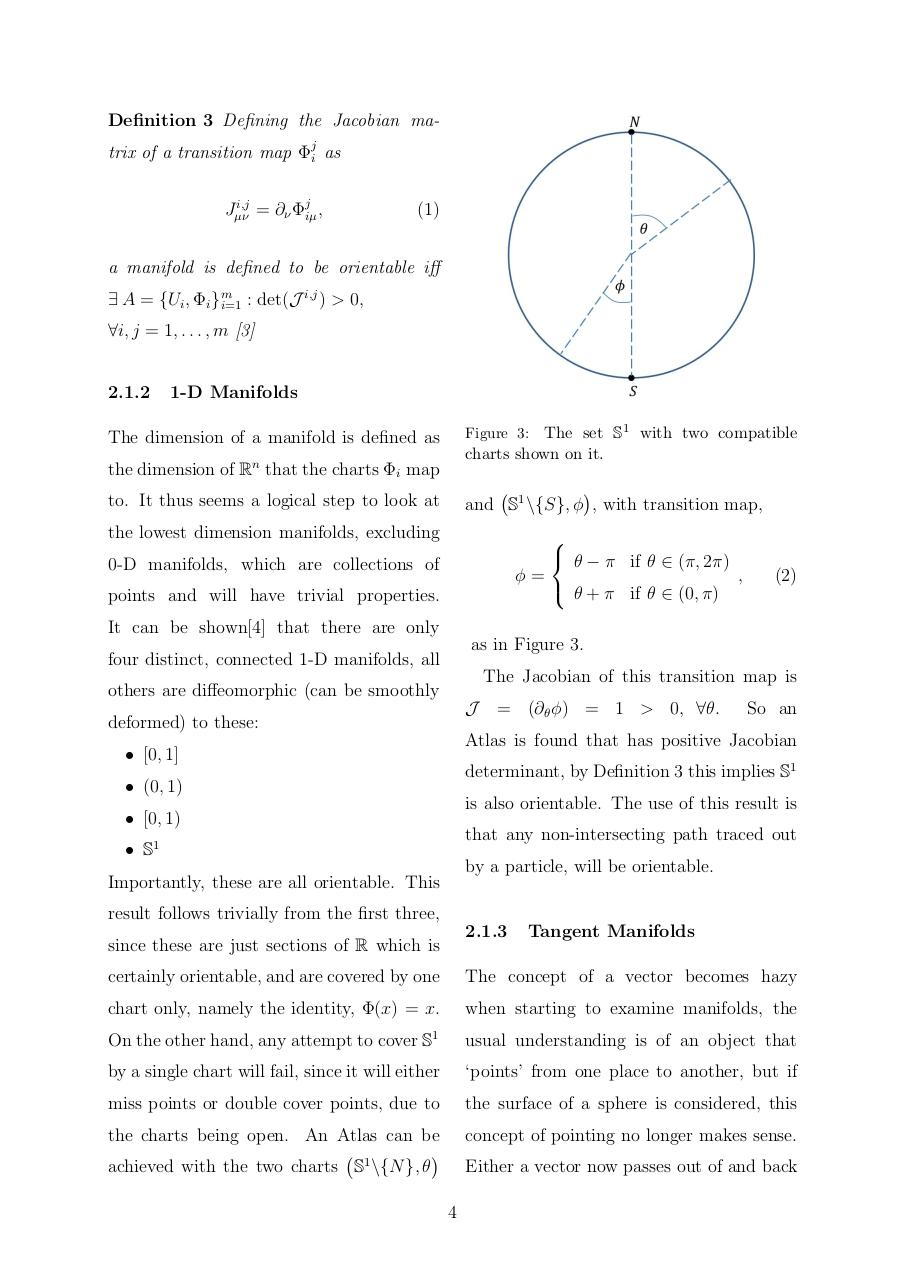# Noether's Theorem on Non Trivial Manifolds By Daniel Martin.pdfPage 1 2 3 45620

#### Text preview

Definition 3 Defining the Jacobian matrix of a transition map Φji as
i,j
Jµν
= ∂ν Φjiµ ,

(1)

a manifold is defined to be orientable iff
i,j
∃ A = {Ui , Φi }m
i=1 : det(J ) &gt; 0,

∀i, j = 1, . . . , m 

2.1.2

1-D Manifolds
Figure 3: The set S1 with two compatible

The dimension of a manifold is defined as

charts shown on it.

the dimension of Rn that the charts Φi map
to. It thus seems a logical step to look at

and S1 \{S}, φ , with transition map,

the lowest dimension manifolds, excluding

 θ − π if θ ∈ (π, 2π)
φ=
,
 θ + π if θ ∈ (0, π)

0-D manifolds, which are collections of
points and will have trivial properties.

(2)

It can be shown that there are only
as in Figure 3.

four distinct, connected 1-D manifolds, all

The Jacobian of this transition map is

others are diffeomorphic (can be smoothly
J

deformed) to these:

= (∂θ φ) = 1 &gt; 0, ∀θ.

So an

Atlas is found that has positive Jacobian

• [0, 1]

determinant, by Definition 3 this implies S1

• (0, 1)

is also orientable. The use of this result is

• [0, 1)

that any non-intersecting path traced out

• S1

by a particle, will be orientable.

Importantly, these are all orientable. This
result follows trivially from the first three,

2.1.3

since these are just sections of R which is

Tangent Manifolds

certainly orientable, and are covered by one

The concept of a vector becomes hazy

chart only, namely the identity, Φ(x) = x.

when starting to examine manifolds, the

On the other hand, any attempt to cover S1

usual understanding is of an object that

by a single chart will fail, since it will either

‘points’ from one place to another, but if

miss points or double cover points, due to

the surface of a sphere is considered, this

the charts being open. An Atlas can be

achieved with the two charts S1 \{N }, θ

concept of pointing no longer makes sense.
Either a vector now passes out of and back
4